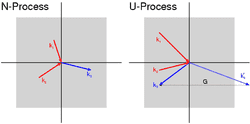# Umklapp scattering

Altruist
what causes this? why is it important? I don't understnad why the phonon momentum is different but the k-vectors outside the first Brillouin zone are physically equivalent to vectors inside it. I can see that physically the vector is shifted by pi back into the Brillouin zone by G. Is the k vector representing momentum of the phonon? if not, what is?n process on the left, u process on the right

Last edited:

bpsbps
First draw a grid of points (2 or 3 points is fine) in 1D separated by the distance a. The grid points are the atoms

Next draw the curve Cos[k*x] for the below k values. Place the atoms on the curve. The oscillation is the displacement of atoms in a transverse lattice vibration.

k=0
k=0.5*pi/a
k=pi/a
k=2*pi/a
k=4*pi/a

Note that:

for k=0 all the atoms are uniformly shifted.

for k=0.5* pi/a it takes two periods for the atoms to be completely out of phase (one all the way up and the other all the way down)

for k=pi/a nearest neighbor atoms are completely out of phase

for k=2*pi/a again all the atoms are uniformly shifted. k=0 and k=2*pi/a are identical

for k=4*pi/a again the same.... and so on for each additional n*2*pi/a (n=0,1,2,3,...)

So k values that are differ by 2*pi/a are identical.

You can test this by plotting Cos[(0.5*pi/a) * x] and Cos[(0.5*pi/a + 2*pi/a) * x].